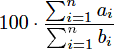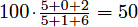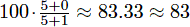# 【POJ2976】Dropping tests - 01分数规划

## Description

In a certain course, you take n tests. If you get ai out of bi questions correct on test i, your cumulative average is defined to be.

Given your test scores and a positive integer k, determine how high you can make your cumulative average if you are allowed to drop any k of your test scores.

Suppose you take 3 tests with scores of 5/5, 0/1, and 2/6. Without dropping any tests, your cumulative average is. However, if you drop the third test, your cumulative average becomes.

## Input

The input test file will contain multiple test cases, each containing exactly three lines. The first line contains two integers, 1 ≤ n ≤ 1000 and 0 ≤ k < n. The second line contains n integers indicating ai for all i. The third line contains n positive integers indicating bi for all i. It is guaranteed that 0 ≤ ai ≤ bi ≤ 1, 000, 000, 000. The end-of-file is marked by a test case with n = k = 0 and should not be processed.

## Output

For each test case, write a single line with the highest cumulative average possible after dropping k of the given test scores. The average should be rounded to the nearest integer.

## 思路

/************************************************
*Author        :  lrj124
*Created Time  :  2018.09.28.20:35
*Mail          :  1584634848@qq.com
*Problem       :  poj2976
************************************************/
#include <algorithm>
#include <iostream>
#include <cstdio>
#include <cmath>
using namespace std;
const int maxn = 1000 + 10;
int n,k,a[maxn],b[maxn];
double tmp[maxn];
inline bool check(double x) {
for (int i = 1;i <= n;i++) tmp[i] = a[i]-x*b[i];
sort(tmp+1,tmp+n+1);
double ans = 0;
for (int i = k+1;i <= n;i++) ans += tmp[i];
return ans >= 0;
}
int main() {
while (cin >> n >> k) {
if (!n && !k) break;
for (int i = 1;i <= n;i++) cin >> a[i];
for (int i = 1;i <= n;i++) cin >> b[i];
double l = 0,r = 0x3f3f3f3f;
while (fabs(r-l) >= 1e-6) {
double mid = (l+r)/2;
if (check(mid)) l = mid;
else r = mid;
}
int ans = int(l*100+0.5);
printf("%d\n",ans);
}
return 0;
}
posted @ 2018-09-28 21:35  lrj124  阅读(177)  评论(0编辑  收藏